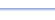Program: NEWTON

NEWTON

• Calculates successive approximations for the zeros of a function, using Newton's Method.

Useful for:

• Calculus

See full list of programs

Example

Q: Use Newton's Method to approximate the zero of the function:

Solution:

Press 'Y=' and enter
the function in Y1:
Remember, always use
'X' as the variable when
using this screen.

Run NEWTON.  How?

Here, we are using the
initial guess as x=4.

Press ENTER.
The program reports
the first approximation
using Newton's Method.

Each time you press
ENTER, the program
performs the next approximation.

The program stops when successive approximations differ by less than 10^-10.  (Depending on your question, you may need to stop sooner.)

Interpretation: 3.83346916